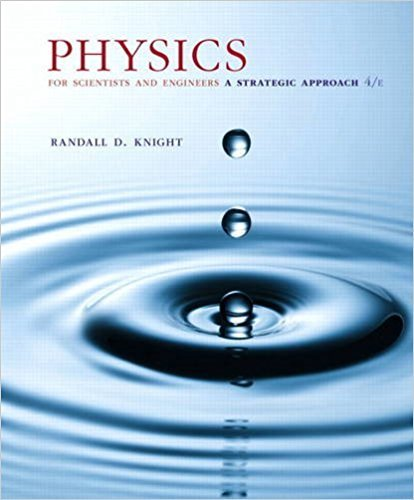×

# You have two containers of equal volume. One is full ofISBN: 9780134081496 191

## Solution for problem 18.17 Chapter 18

Physics for Scientists and Engineers: A Strategic Approach, Standard Edition (Chs 1-36) | 4th Edition

• Textbook Solutions
• 2901 Step-by-step solutions solved by professors and subject experts
• Get 24/7 help from StudySoup virtual teaching assistantsPhysics for Scientists and Engineers: A Strategic Approach, Standard Edition (Chs 1-36) | 4th Edition

4 5 0 345 Reviews
28
4
Problem 18.17

You have two containers of equal volume. One is full of helium gas. The other holds an equal mass of nitrogen gas. Both gases have the same pressure. How does the temperature of the helium compare to the temperature of the nitrogen? a. Thelium 7 Tnitrogen b. Thelium = Tnitrogen c. Thelium 6 Tnitrogen

Step-by-Step Solution:
Step 1 of 3

2 1 14 0 2.10 140 R3.90 B B...

Step 2 of 3

Step 3 of 3

##### ISBN: 9780134081496

This full solution covers the following key subjects: . This expansive textbook survival guide covers 42 chapters, and 4463 solutions. Physics for Scientists and Engineers: A Strategic Approach, Standard Edition (Chs 1-36) was written by and is associated to the ISBN: 9780134081496. The answer to “You have two containers of equal volume. One is full of helium gas. The other holds an equal mass of nitrogen gas. Both gases have the same pressure. How does the temperature of the helium compare to the temperature of the nitrogen? a. Thelium 7 Tnitrogen b. Thelium = Tnitrogen c. Thelium 6 Tnitrogen” is broken down into a number of easy to follow steps, and 54 words. The full step-by-step solution to problem: 18.17 from chapter: 18 was answered by , our top Physics solution expert on 12/28/17, 08:06PM. Since the solution to 18.17 from 18 chapter was answered, more than 228 students have viewed the full step-by-step answer. This textbook survival guide was created for the textbook: Physics for Scientists and Engineers: A Strategic Approach, Standard Edition (Chs 1-36), edition: 4.

Unlock Textbook Solution

You have two containers of equal volume. One is full of

×